Home > CC2 > Chapter 8 > Lesson 8.3.4 > Problem8-98

8-98.

You learned in an earlier lesson how to classify an angle by comparing it to a 90º right angle. Find the measure of each angle below and classify it according to its measure. Homework Help ✎

 a. What is the measure of half of a right angle?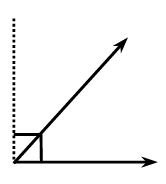b. If the angle at right is made with a right angle added to half of a right angle, what is its measure?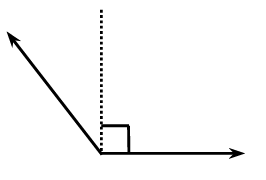c. Suppose a right angle is divided into three equal angles as shown at right. What is the measure of each angle?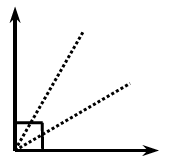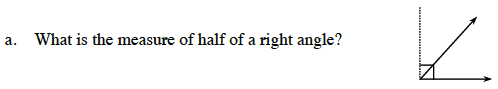Remember that a right angle is 90°.
Thus, to find the measure of half a right angle, we must divide 90° by 2.

45°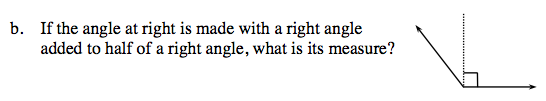In part (a), you found the measure of half a right angle.
Now you must add your answer from part (a) to 90° to find the measure of a right angle added to half a right angle.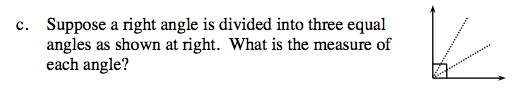Similar to part (a), you must divide 90° by 3 to find the measure of a third of a right angle.